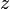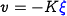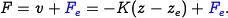# Errata: In Example 6.8, the state error should be \xi and the input should be F

Location: page 192, line 4-14

The symbolrepresents both the system state and the state error, and the symbolshould be the input forces. The correct text should readIt can be verified that the system is reachable.

To compute a linear quadratic regulator for the system, we write the cost function aswhereandrepresent the local coordinates around the desired equilibrium point. We begin with diagonal matrices for the state and input costs:This gives a control law of the form, which can then be used to derive the control law in terms of the original variables:As computed in Example 5.4, the equilibrium points haveand.

(Contributed by C.-H. Choi, 7 Dec 2010)